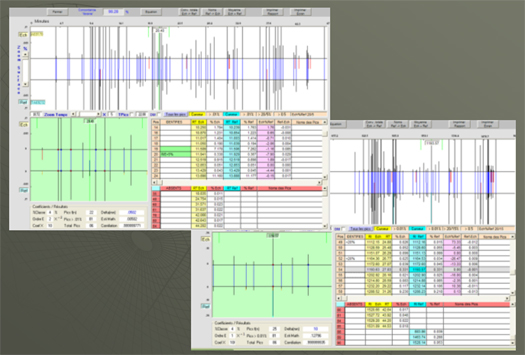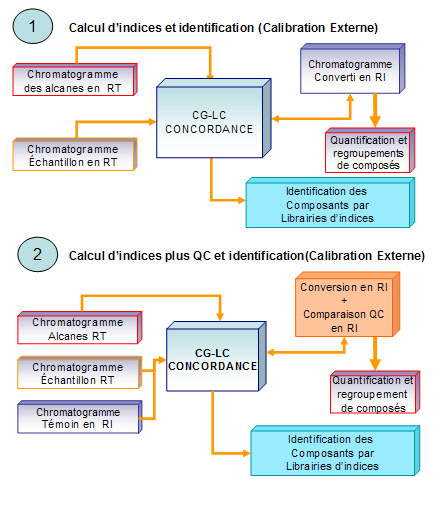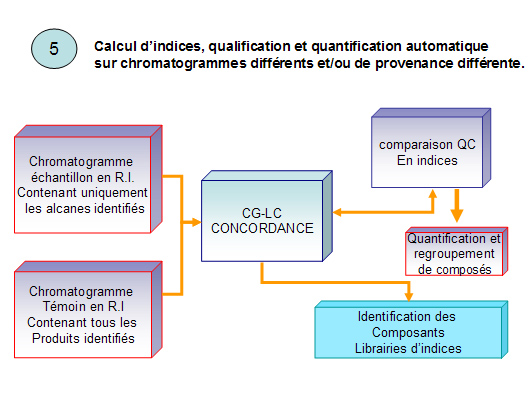info@spectrochrom.com

00353 857087743# Conv. RT / RI (Kovats index)

Working directly with retention indices helps to standardize the eluates for a specific column (phase). Each product is associated with a retention index predetermined by a calibration with linear hydrocarbons, alkanes with CnH2n+2 formula.

This conversion is made using two formulas defined by Kovats in 1958, the first one in isotherm, the second one for temperature settings (Linear Kovats Retention Index).

Tr (p) retention time (tr) of the product in between retention time of alkanes tr (n) and tr (n+1)

(Example with n = 1 & 120)

• Ip(iso) = 100*(((log tr(p) – log tr(n)) / ( log tr(n+1) – log tr(n))) + 100* n (I.R. Kovats)
• Ip(pt°) = 100*(((tr(p) – tr(n)) / (tr(n+1)- tr(n))) + 100* n (I.R. linéaire)## 5 ways to work with retention indices